### Exercise 1:

Construct the projections of a right square pyramid. The axis of the pyramid lies on the line $$\small o[O_1(-1,13,0); P(15,1.5,14.5)]\,\,$$, the point $$\small A(6,3,3)$$ is a vertex of the base and the height of the pyramid equals $$\small v=9$$.

Scheme of spatial solution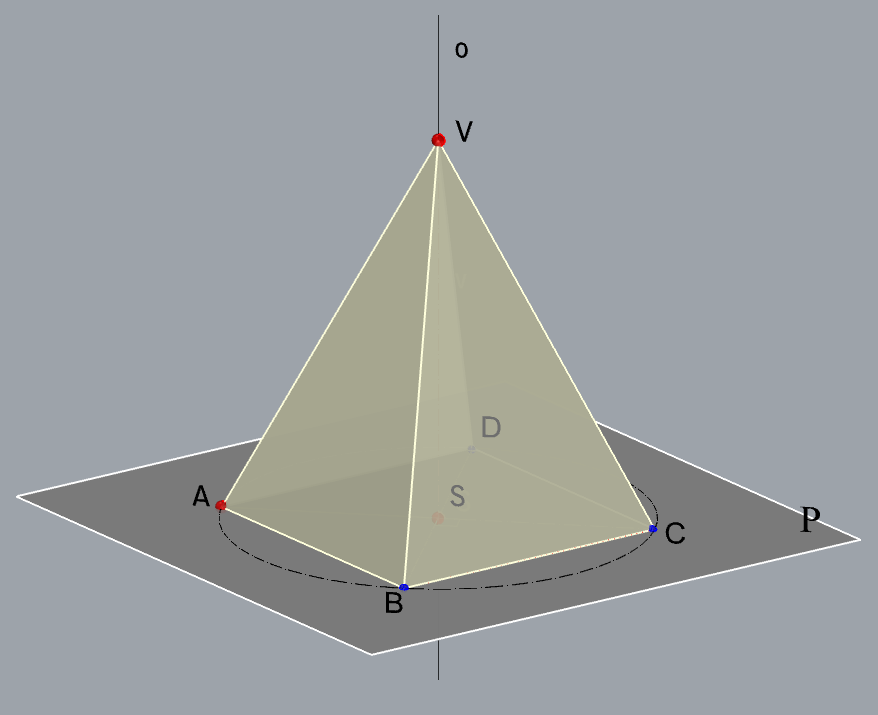$$\small\mathrm P$$ $$\small -$$ plane of the base$$\small A\in\,$$$$\small\mathrm P$$, $$\small o \perp$$ $$\small\mathrm P$$ $$\small S-$$ center of the base$$\small S=o\cap$$ $$\small\mathrm P$$ $$\small \Box ABCD\subset\,\,$$$$\small\mathrm P$$ $$\small V-$$ the apex$$\small V\in o$$, $$\small \,\,d(S,V)=v$$

Solution via Monge's method (step by step)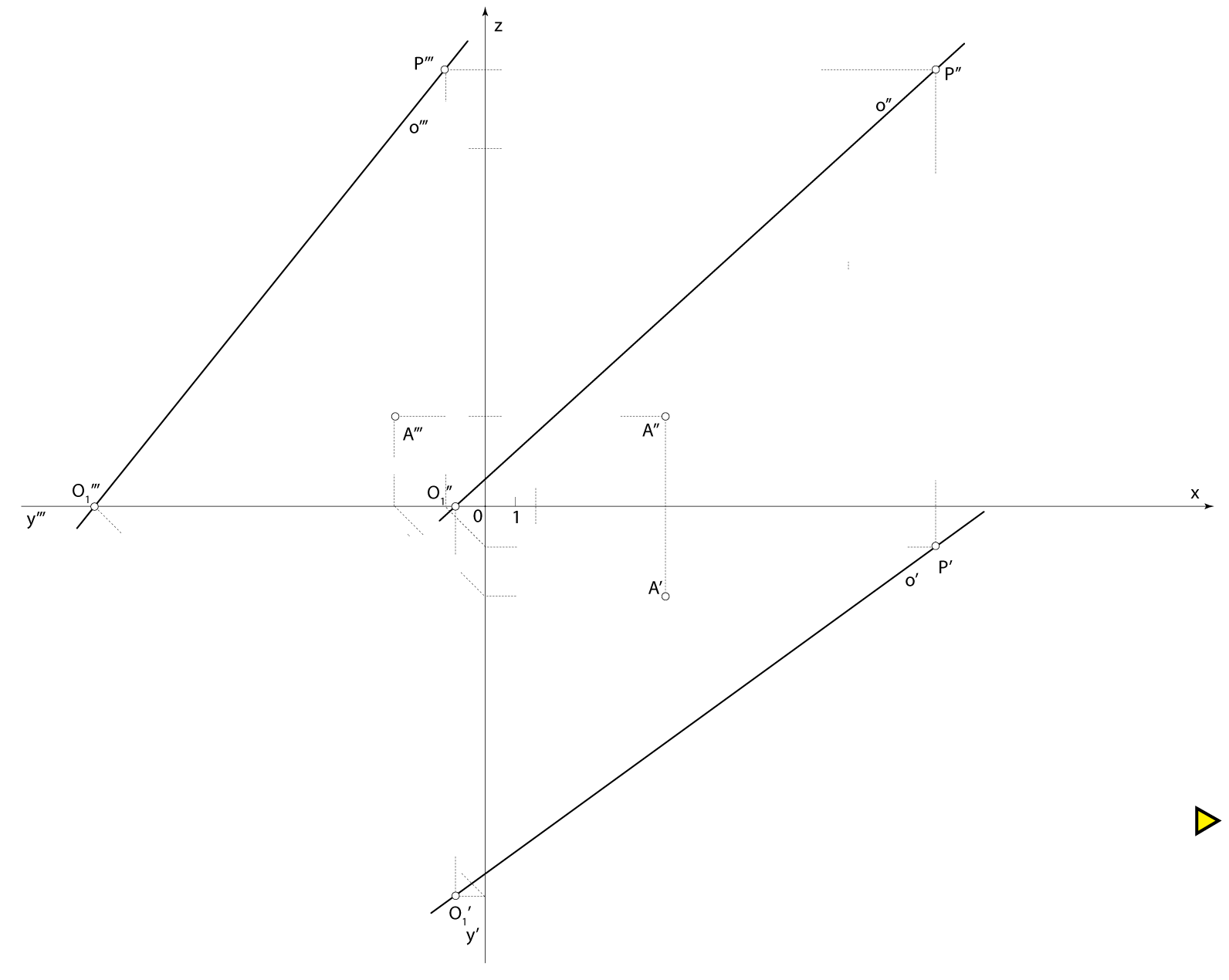Final drawing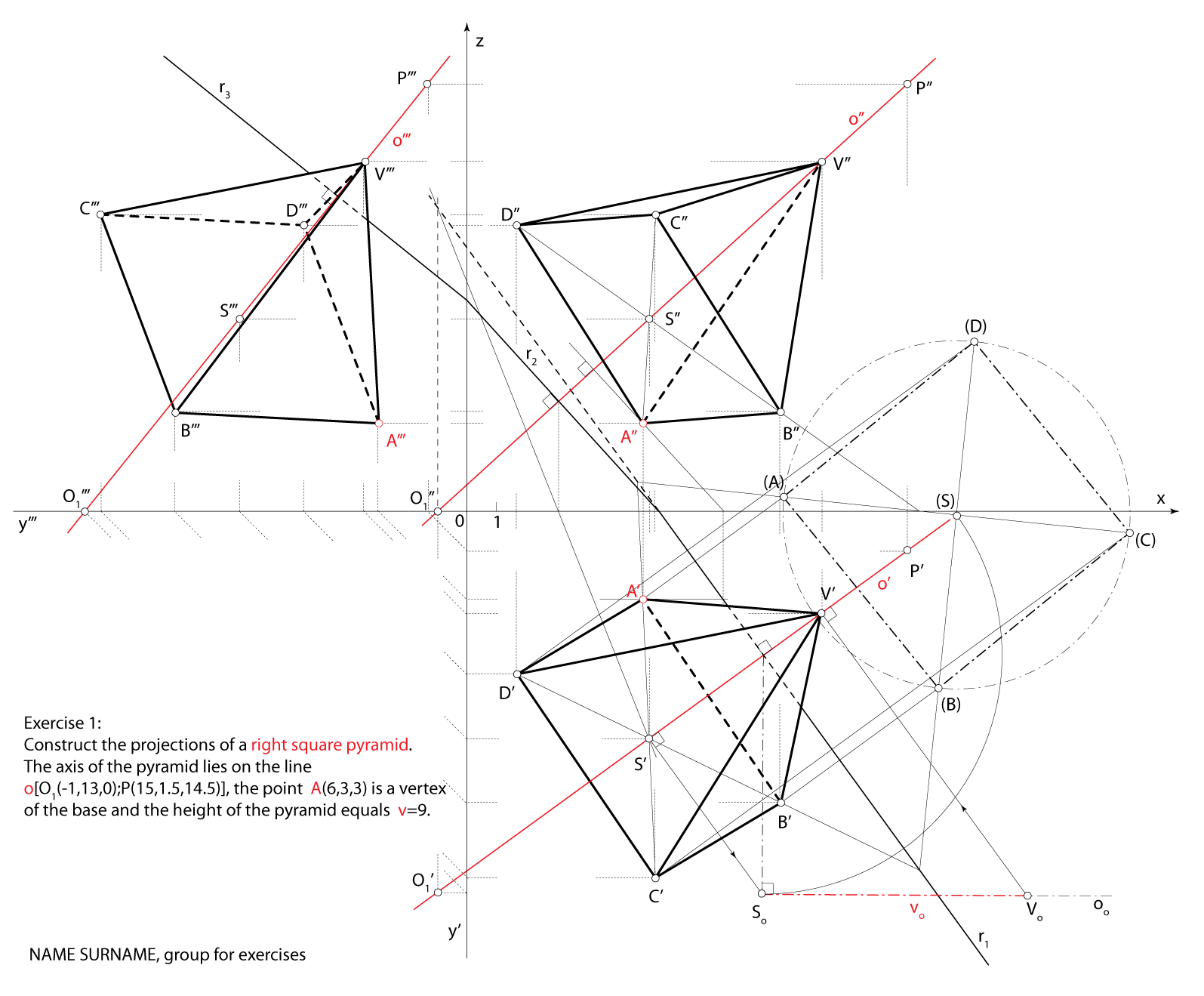### Exercise 2:

Construct the projections of a cube if one edge of the cube lies on the line $$\small p[P_1(3,6,0); P_2(20,0,12)]$$ and one vertex that is on the same side as the given edge is the point $$\small A(16,7,4)$$.

Scheme of spatial solution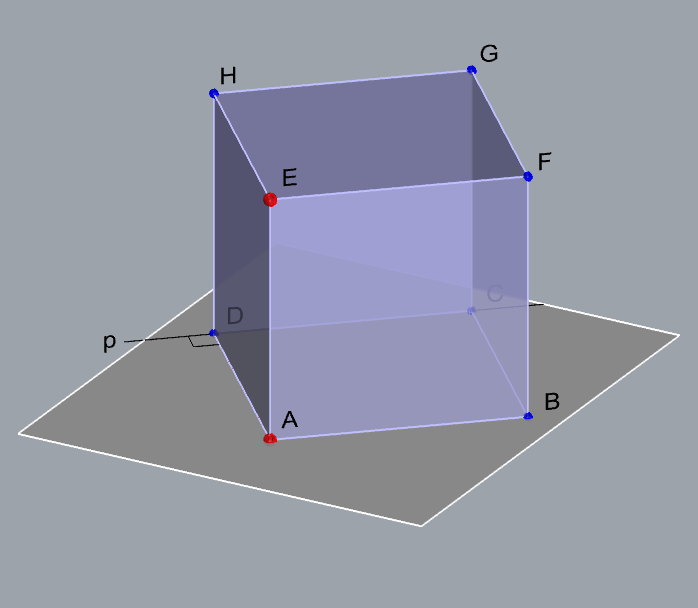$$\small\mathrm P$$ $$\small -$$ plane that contains one side of the cube$$\small A\in$$ $$\small\mathrm P$$, $$\small p \subset$$ $$\small\mathrm P$$ $$\small \overline{AD}-$$ one edge of the cube$$\small D\in p$$, $$\small \overline{AD}\perp p$$ $$\small \Box ABCD\subset\,\,$$$$\small\mathrm P$$ $$\small E-$$ a vertex of the cube lying in a plane parallelto the plane $$\small\mathrm P$$$$\small \overline{AE}\perp\,\,$$$$\small\mathrm P$$, $$\small \,\,d(A,E)=d(A,D)$$ $$\small \Box EFGH$$ in a plane parallel to $$\small\mathrm P$$

Solution via Monge's method (step by step)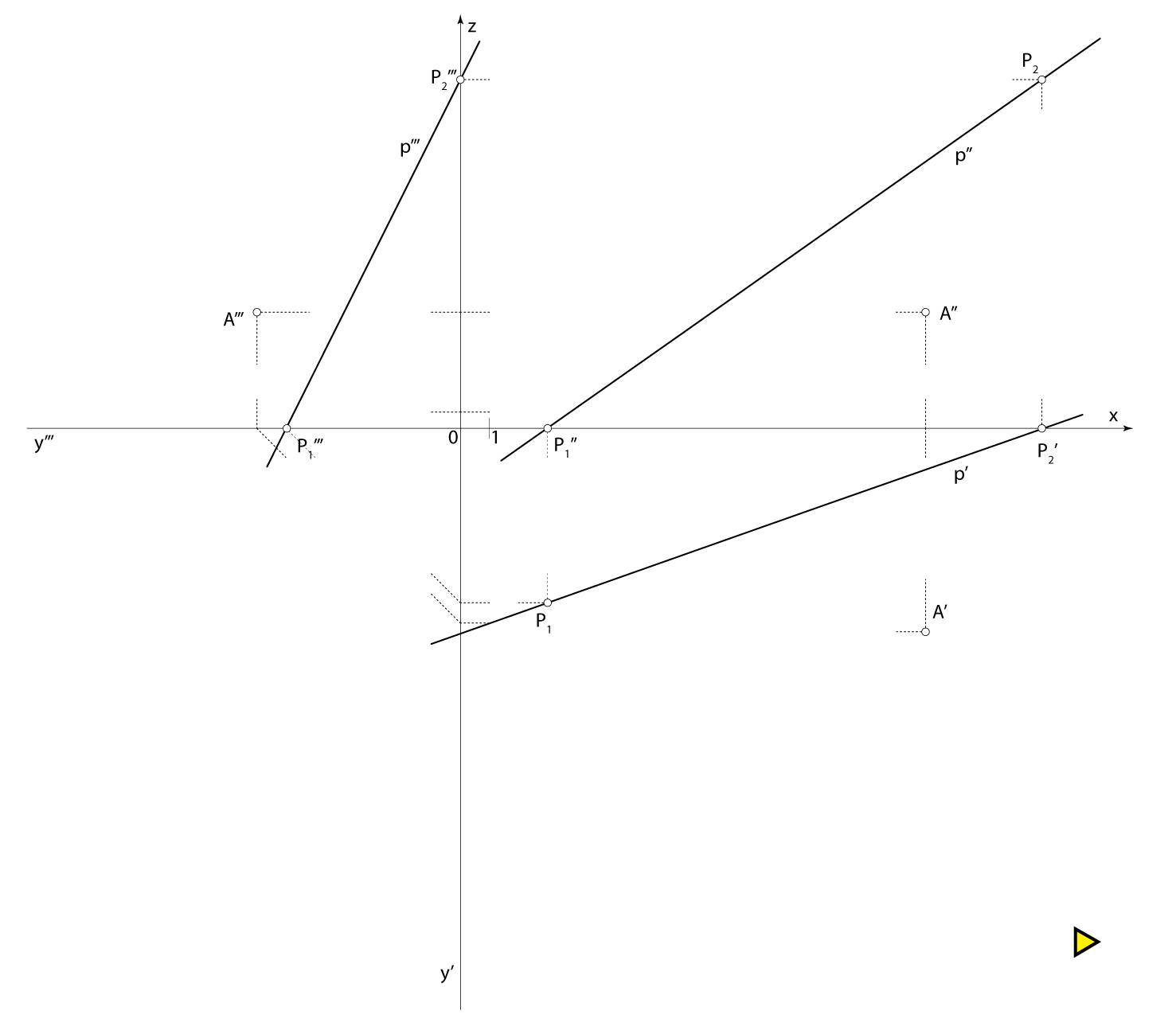Final drawing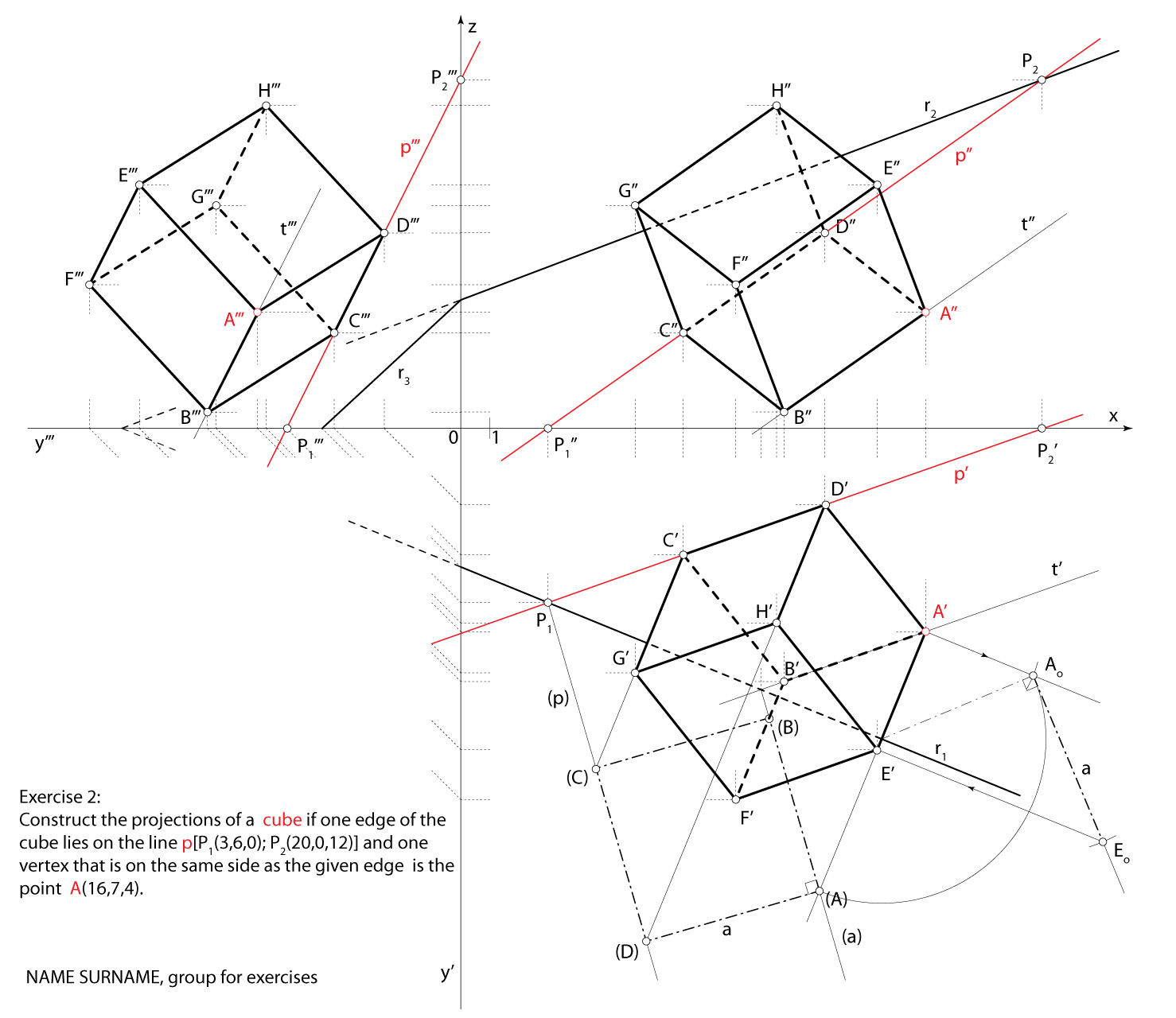### Exercise 3:

Construct the projections of a cone of revolution with the vertex $$\small V(2,10,1)$$, the axis lying on the line $$\small o [V, O_1(1,11.5,0)]$$, one generatrix lying on the line $$\small i [V, I_1(-1,12.5,0)]$$ and the radius of the base equal to $$\small r=3.5$$.

Scheme of spatial solution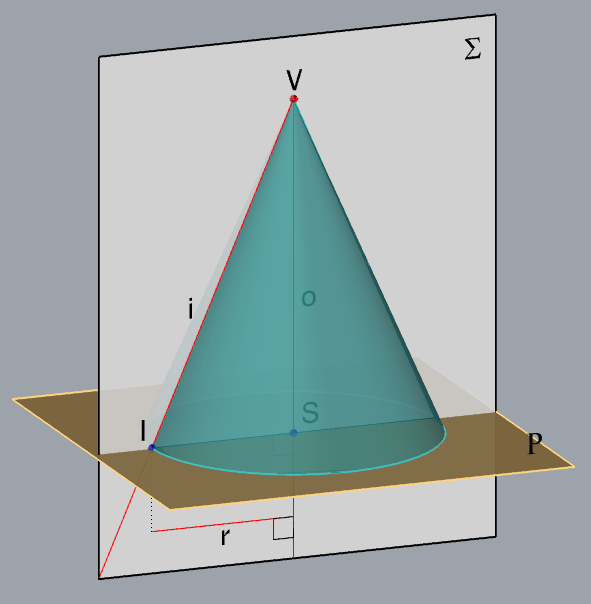$$\small \Sigma$$ $$\small -$$ axial cross-section plane$$\small o\subset \Sigma$$, $$\small i\subset \Sigma$$ $$\small \triangle ISV \subset \Sigma$$$$\small S-$$ center of the base$$\small I -$$ foot of the generatrix $$\small i$$ $$\small\mathrm P$$ $$\small -$$ plane of the base$$\small S\in$$ $$\small\mathrm P$$, $$\small o \perp$$ $$\small\mathrm P$$ A circle in the plane $$\small\mathrm P$$ with center $$\small S$$ andradius $$\small \overline{SI}$$ is the base circle of the given cone.

Solution via Monge's method (step by step)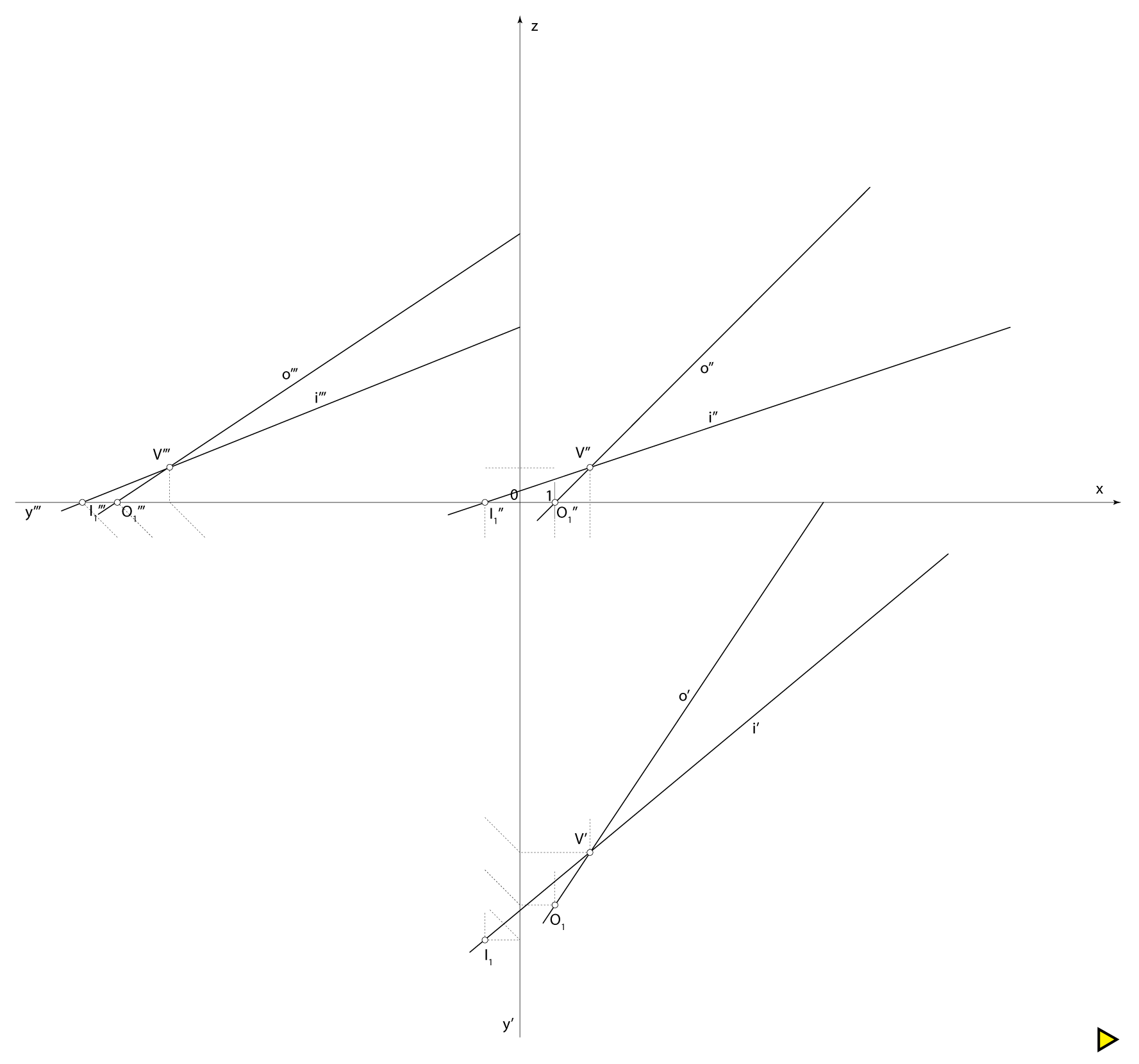Final drawing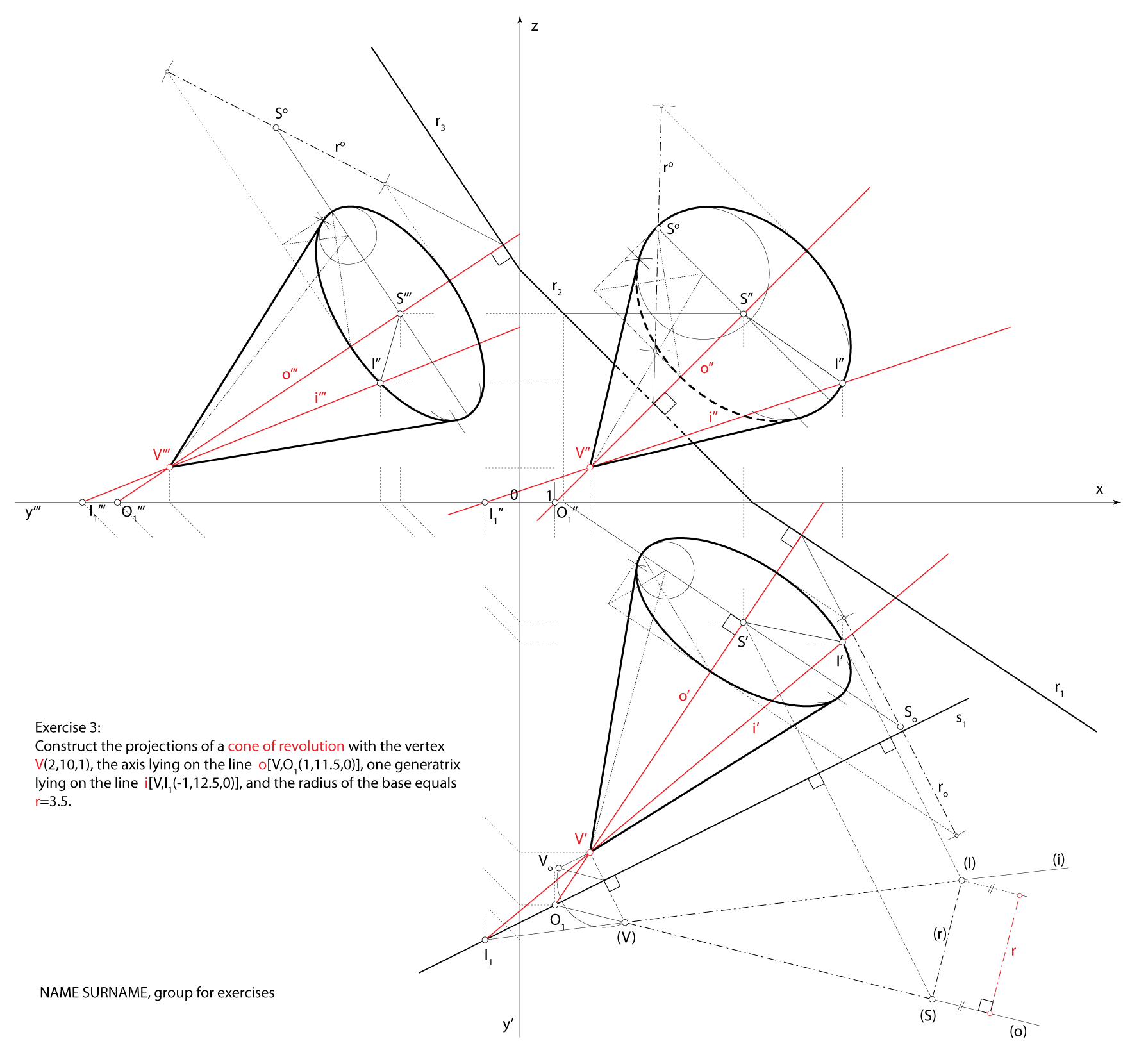### Exercise 4:

Construct the projections of a cone of revolution with given vertex $$\small V(6,14,12)$$ whose base lies in the plane $$\small\mathrm P$$$$\small (4,-3,-5)$$ and touches the plane $$\small \Pi_2$$.

Scheme of spatial solution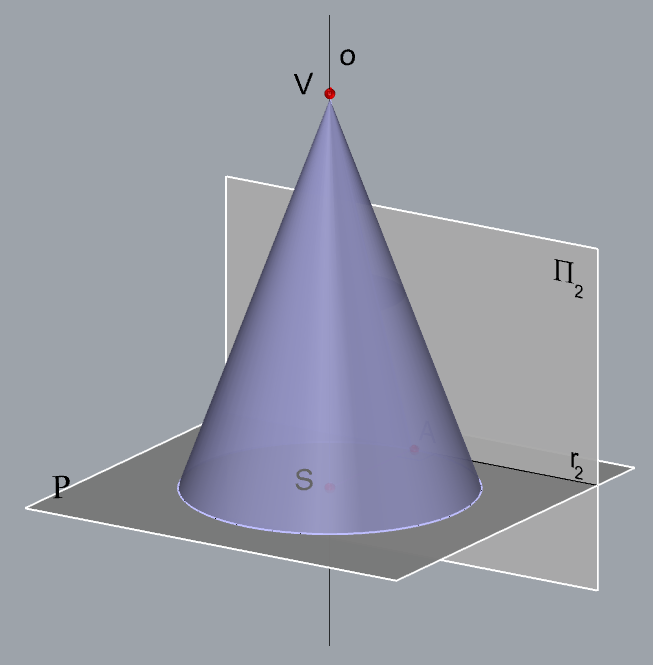$$\small o \,-$$ axis of the cone$$\small V\in o$$, $$\small o \perp\,$$ $$\small\mathrm P$$ $$\small S \,-$$ center of the base$$\small S=o\cap\,$$$$\small\mathrm P$$ $$\small A \,-$$ point on the base circle$$\small A\in r_2$$, $$\small SA\,\perp\,r_2$$ A circle in the plane $$\small\mathrm P$$ with center $$\small S$$ and radius$$\small \overline{SA}$$ is the base of the given cone.

Solution via Monge's method (step by step)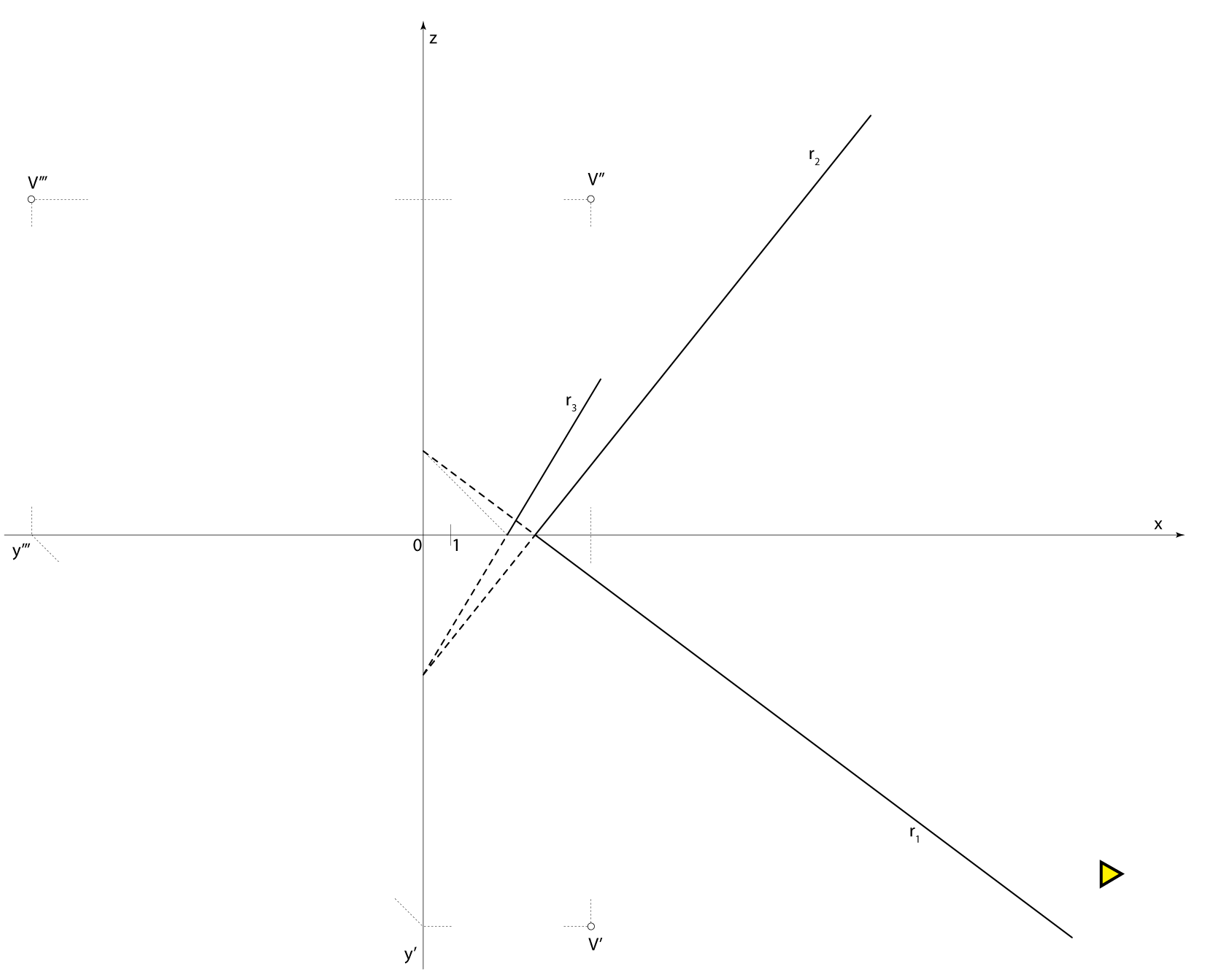Final drawing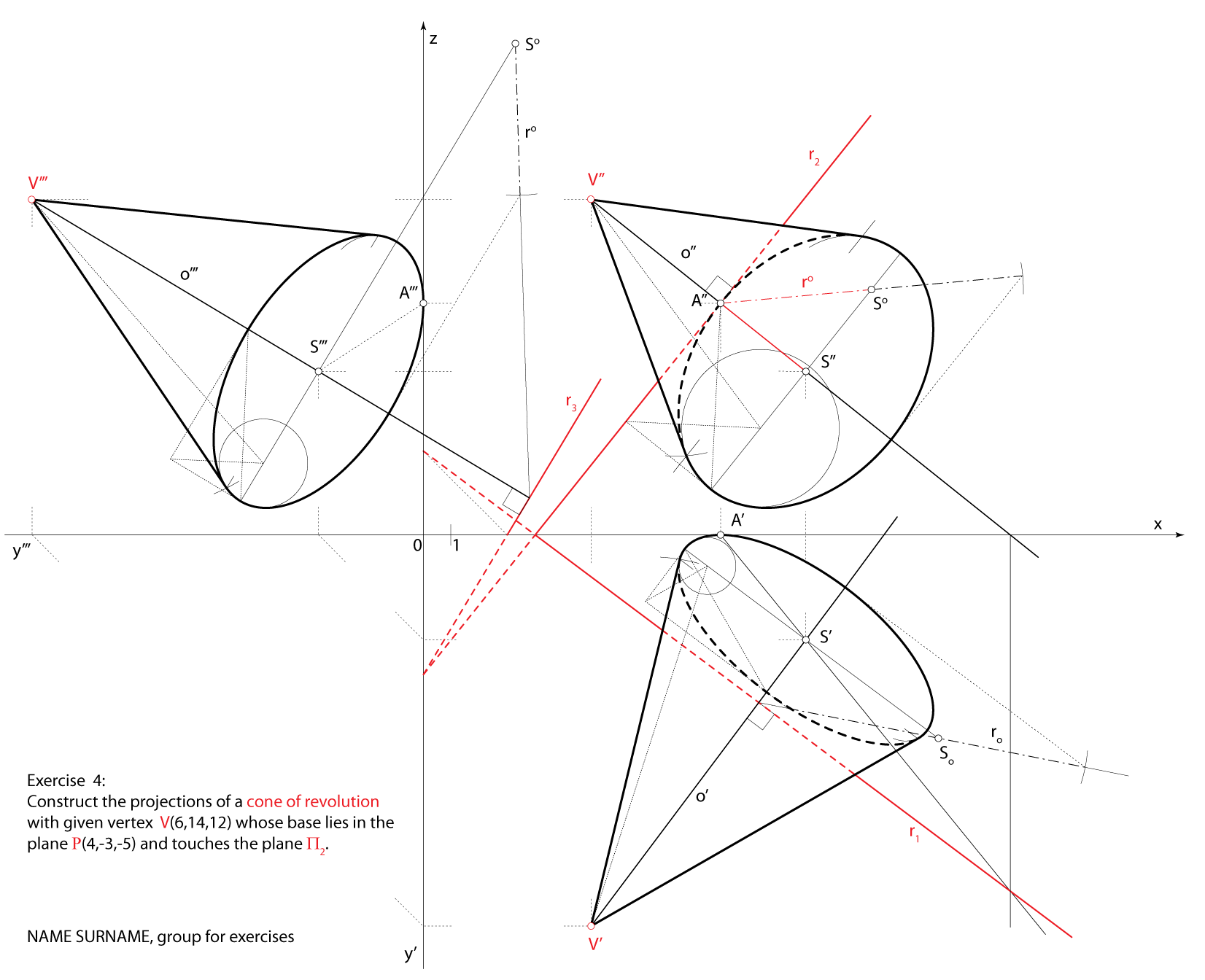### Exercise 5:

Construct the projections of a cylinder of revolution if the segment $$\small \overline{GH}[G(11,11,4);H(15,13,6)]$$ is a chord of one base, the axis lies in the plane $$\small \Gamma(2,-1.5,-6)$$ and the height of the cylinder is $$\small v=10.5$$.

Scheme of spatial solution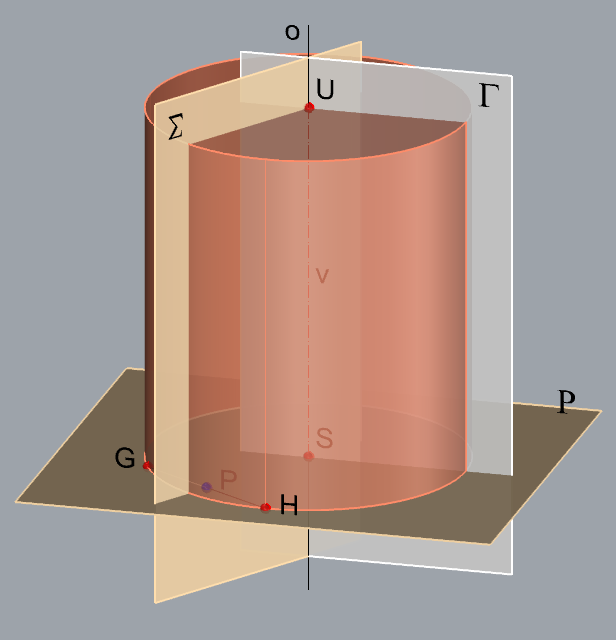$$\small \Sigma -$$ bisector plane of the line segment $$\small \overline {GH}$$$$\small P -$$ midpoint of the line segment $$\small \overline {GH}$$,$$\small P \in \Sigma$$, $$\small \overline {GH} \perp \Sigma$$ $$\small o -$$ axis of the cylinder, $$\small o = \Sigma \cap \Gamma$$ $$\small\mathrm P$$$$\small -$$ plane of one base, $$\small G\in$$ $$\small\mathrm P$$, $$\small o \perp$$ $$\small\mathrm P$$ $$\small S -$$ center of the base, $$\small S = o \cap$$ $$\small\mathrm P$$ The cirle of the base lies in the plane $$\small\mathrm P$$and the radius is $$\small \overline {SG}$$. $$\small U -$$ center of the other base$$\small U \in o$$, $$\small d(S,U)=v$$.

Solution via Monge's method (step by step)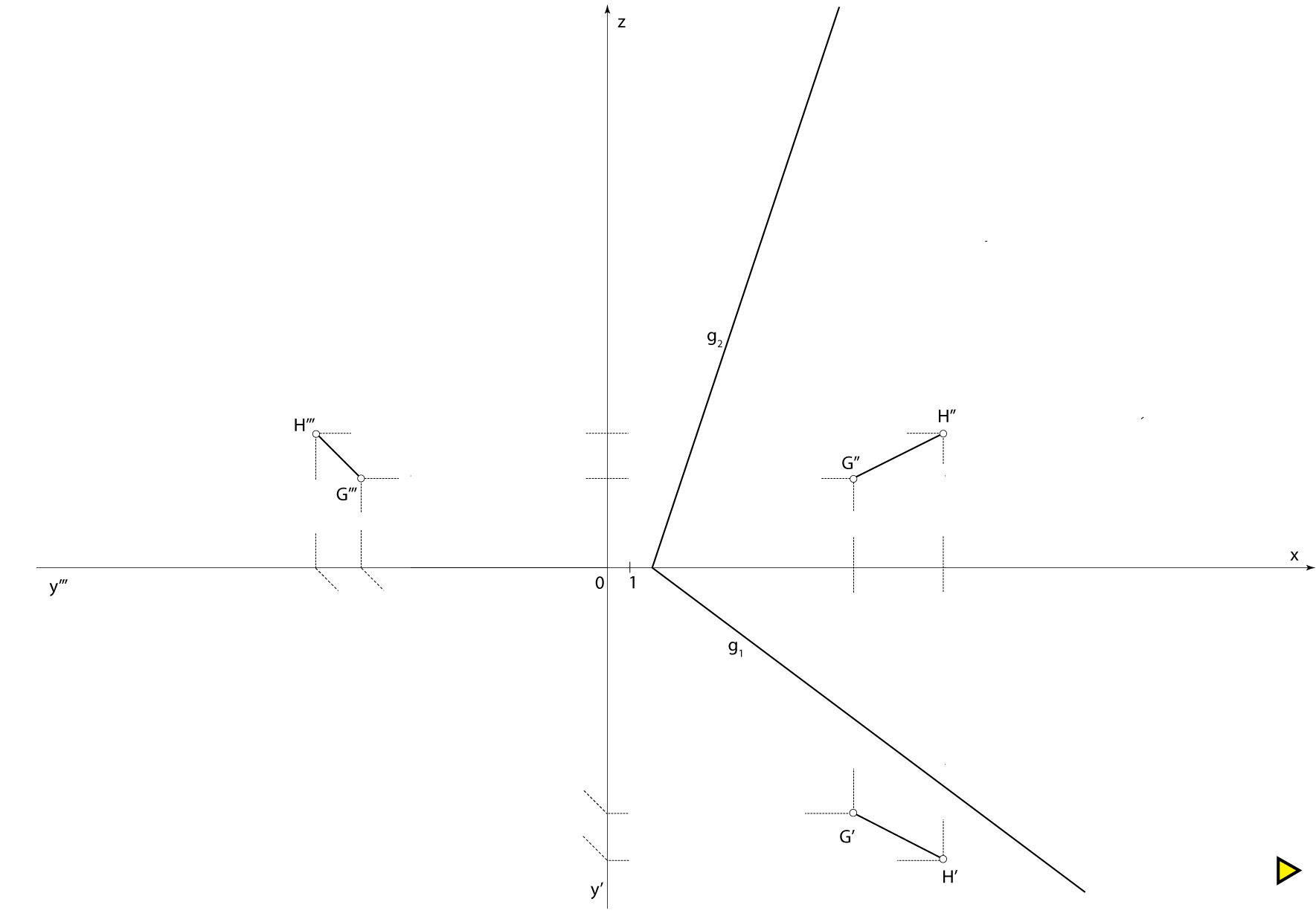Final drawing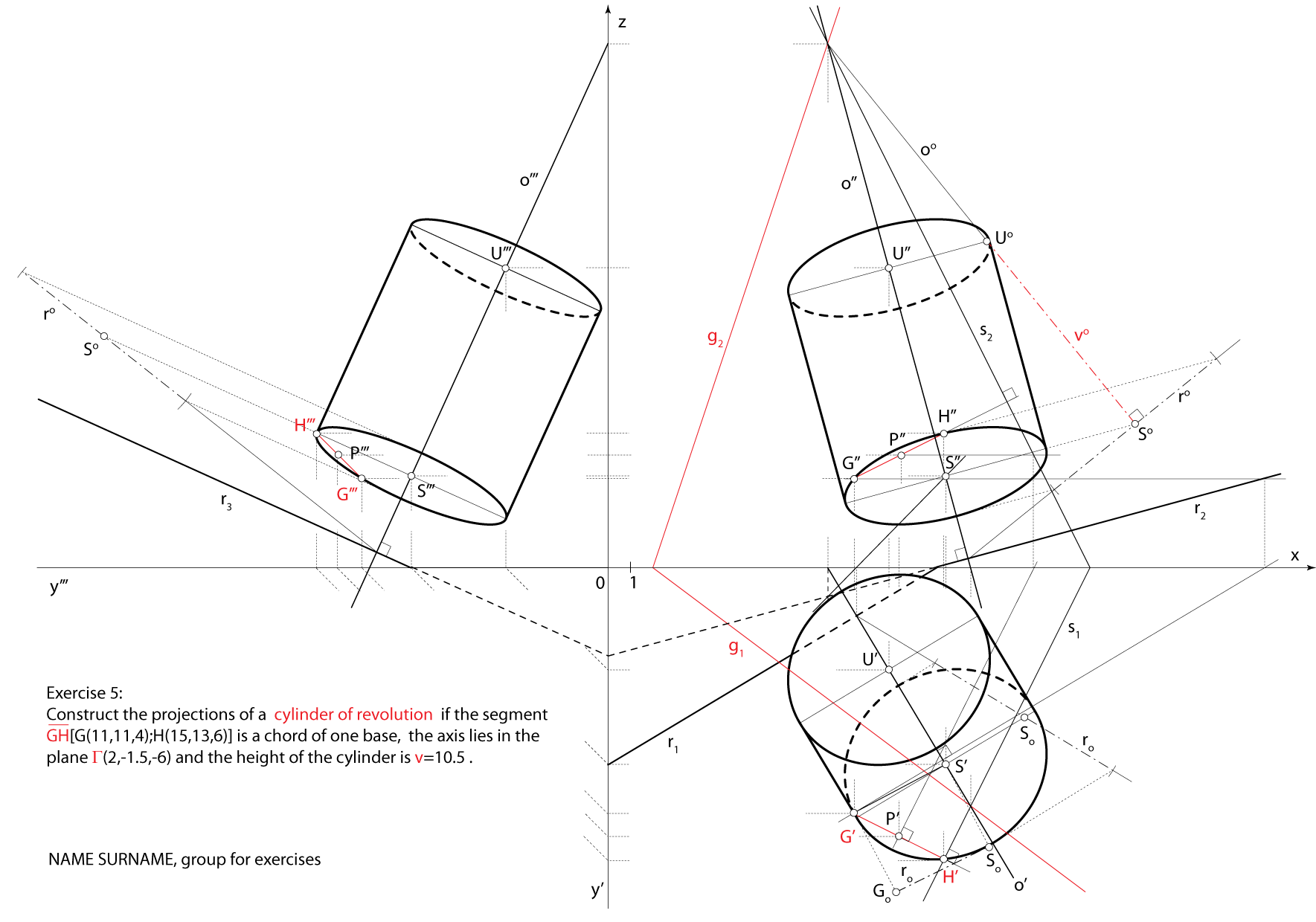Created by Sonja Gorjanc, translated by Helena Halas and Iva Kodrnja